# HINT: A New Way To Measure Computer Performance*

## Technical Description of HINT

### Figures

Figure 1. Area to be bounded by HINT.
Figure 2. Integration with byte-precision numbers, two subintervals.
Figure 3. Sequence of hierarchical refinement of integral bounds.
Figure 4. Presicion-limited last iteration, 8-bit data.
Figure 5. Comparison of different precisions.
Figure 6. Comparison of various workstations.
Figure 7. Comparison of several parallel systems.

## Abstract

The computing community has long faced the problem of scientifically comparing different computers and different algorithms. When architecture, method, precision, or storage capacity is very different, it is difficult or misleading to compare speeds using the ratio of execution times. We present a practical and fair approach that provides mathematically sound comparison of computational performance even when the algorithm, computer, and precision are changed. HINT removes the need for pseudo-work measures such as "Mflop/s" or "MIPS." It reveals memory bandwidth and memory regimes, and runs on any memory size. The scalability of HINT allows it to compare computing as slow as hand calculation to computing as fast as the largest supercomputers. It ports to every sequential and parallel programming environment with very little effort, permitting fair but low-cost comparison of any architecture capable of digital arithmetic.

## 1. Introduction

From the days of the first digital computers to about the mid-1970s, comparing computer performance was not the headache it is now. Most computers presented the user with the appearance of the von Neumann model of a single instruction stream and a single memory, and took much longer for floating-point operations than for other operations. Thus, an algorithm with fewer floating-point operations (flop) than another in its sequential description could be safely assumed to run in less time on a given computer. It also meant that a computer with a higher rated capability of flop/s would almost certainly run a given (same size) algorithm in less time. The model wasn't linear (halving the operations or doubling the nominal computer flop/s didn't exactly halve the execution time), but at least it made predictions that were usually in the right direction.

It doesn't work anymore. Most algorithms do more data motion than arithmetic, and most current computers are limited by their ability to move data, not to do arithmetic. While there has been much hand-wringing over misreporting of performance results , there has not been a constructive proposal of what should be done instead. Scientists and engineers express surprise and frustration at the increasing rift between nominal speed (as determined by nominal MIPS or Mflop/s) and actual speed for their applications. Use of memory bandwidth figures in Mbytes/s is too simplistic because each memory regime (registers, primary cache, secondary cache, main memory, disk, etc.) has its own size and speed; parallel memories compound the problem.

### 1.1 The failure of other "speed" measures

The SPEC benchmark [3, 11] is popular among workstation vendors. It is not an independent measure; a consortium of vendors determine what is in SPEC and how to report it. SPEC does not scale, and runs on a narrow range of computers at any given time. It has had to be revised once, as the first version proved too small for workstations after a few years of technological progress. SPEC claims to be the geometric ratio of the time reduction of various kernels and applications to the time required by a VAX-11/780. Unfortunately, the VAX-11/780 currently gets a SPECmark of about 3, indicating it is three times as fast as itself! SPEC survives largely because of the lack of credible alternatives.

The PERFECT Benchmark suite , introduced in the 1980s, has over 100,000 lines of semi-standard Fortran 77 intended to predict application performance by timing sample scientific applications. It has faded almost completely out of sight because it makes benchmarking more difficult than converting the target application and running it. PERFECT benchmark figures are only available for a handful of computer systems.

Snelling  has explained how traditional measures of scientific computer performance have little resemblance to measures we use in every other field of human endeavor. Scientists used to the hard currency of "meters per second" or "reaction rate" are at a loss when they attempt a scientific paper on the performance of their computing method. The only well-defined thing they can measure is time, so they fix the problem being run and measure the run time for various numbers of processors or different types of computers. We agree that speed is work divided by time, but without a rigorous definition of "work," the approach has been to try to keep the work "constant" by fixing the program and using relative speeds. Dividing one speed by another cancels the numerator and leaves a ratio of times, avoiding the need to define "work."

Fixing the program is fallacious, however, since increased performance is used to attack larger problems or reach better quality answers. Whatever the time users are willing to wait, they will scale the job asked of the computer to fit that time. Contrary to the "speedup" studies done in many papers on parallel processing, one does not purchase a thousand-processor system to do the same job as a one-processor system but in one thousandth the time.

We are therefore faced with having to define a numerator for "computational speed." In the past, "Logical Inferences Per Second" has been proposed for artificial intelligence, but there is no such thing as a unit standard logical inference. "VAX unit of performance" has been used by those who would make a popular minicomputer from 1977 a baseline for comparison, but as the SPECmark shows, that standard can vary by at least a factor of three for a variety of reasons. What about Mflop/s? There is no standard "floating-point operation," since different computers expend different relative effort for square roots, absolute values, exponentiation, etc. with varying mantissa lengths and varying amounts of error trappingÉ even within the IEEE Floating Point Standard. Mflop/s numbers do not measure how much progress was made on a computation; they only measure what was done, useful or otherwise. It is analogous to measuring the speed of a human runner by counting footsteps per second, ignoring whether those footsteps covered any distance toward the goal.

If one reads advertising for personal computers, one sees "MHz" as the universal indicator of speed. Buyers have been led to believe that a 66 MHz computer is always faster than a 40 MHz computer, even if the memory and hard disk speed are such that the 66 MHz computer does far less in every clock cycle than the 40 MHz machine. This is like a car advertisement noting only the largest number that appears on the speedometer, and asking the buyer to infer proportional net performance.

Is there any hope, then, for a definition of computational "work"? We feel there is, if one defines the quality of an answer. In Section 2, we define Quality Improvement Per Second (QUIPS) as an example of a measure based rigorously on progress toward solving a problem.

### 1.2. The precedent of SLALOM

SLALOM  was the first benchmark to attempt use of answer quality as the figure of merit. It fixed the time for a radiosity calculation at one minute, and asked how accurately the answer could be calculated in that time. Thus, any algorithm and any architecture could be used, and precision was specified only for the answer file, not for the means of calculating. SLALOM was quickly embraced by the vendor community , because for the first time a comparison method scaled the problem to the power available and permitted each computer to show its application-solving capability. However, SLALOM had some defects:

1. The answer quality measure was simply "patches," the number of areas into which the geometry is subdivided; this measures discretization error only roughly, and ignores roundoff error and solution convergence.

2. The complexity of SLALOM was initially order N3, where N is the number of patches. Published algorithmic advances reduced this to order N2, but it is still not possible to say that a computer that does 2N patches in one minute is "twice as powerful" as one that does N patches in one minute. An order N log N method has been found that does much to alleviate the problem, but it leads to Defect 3:

3. Benchmarks trade ease-of-use with fidelity to real-world problems. Ease-of-use for a benchmark, especially one intended for parallel computers, tends to decrease with lines of code in a serial version of the program. SLALOM started with 1000 lines of Fortran or C, but expanded with better algorithms to about 8000 lines. Parallelizing the latest N log N algorithm has proved expensive; a graduate student took a year to convert it to a distributed memory system, and only got twice the performance of our best workstation. To be useful, a benchmark should be very easy to convert to any computer. Otherwise, one should simply convert the target application and ignore "benchmarks."

4. SLALOM was unrealistically forgiving of machines with inadequate memory bandwidth, especially in its original LINPACK-like form. While this made it popular with computer companies that had optimized their architectures to matrix-matrix operations, it reduced its correlation with mainstream scientific computing, and hence its predictive value.

5. While SLALOM had storage demands that scaled with the computer speed, it failed to run for the required one minute on computers with insufficient memory relative to arithmetic speed. Conversely, computers with capacious memory could not exercise it using SLALOM. Yet memory size is critical to application "performance" in the sense of what one is able to compute, if not in the sense of speed.

## 2. The HINT benchmark

### 2.1 Definition and example

Except for SLALOM and the TPC/A and TPC/B database benchmarks , extant benchmarks are based on the idea of measuring the time various computers take to complete a fixed-size task. The SLALOM benchmark fixes the time at one minute and uses the job size as the figure of merit. The TPC benchmarks scale similarly to the power available, measuring transactions per second for a database that grows depending on the speed of the system being measured.

The HINT benchmark is based on a fundamentally different concept. HINT stands for Hierarchical INTegration. It produces a speed measure we call QUIPS, for Quality Improvement Per Second. HINT fixes neither time nor problem size. Here is an English description of the task measured by HINT:

Use interval subdivision to find rational bounds on the area in the xy plane for which x ranges from 0 to 1 and y ranges from 0 to (1- x) / (1+ x). Subdivide x and y ranges into an integer power of two equal subintervals and count the squares thus defined that are completely inside the area (lower bound) or completely contain the area (upper bound). Use the knowledge that the function (1- x) / (1+ x) is monotone decreasing, so the upper bound comes from the left function value and the lower bound from the right function value on any subinterval. No other knowledge about the function may be used. The objective is to obtain the highest quality answer in the least time, for as large a range of times as possible.

Quality is the reciprocal of the difference between the upper and lower bounds. Timing begins on entry to the program that performs the task; quality increases as a step function of time whenever an improvement to answer quality is computed. Maintain a queue of intervals in memory to split, and try to split the intervals in order of largest removable error. The amount of error removable by further subdivision must be calculated exactly whenever an interval is subdivided. Sort the resulting smaller errors into the last two entries in the queue. The subdivisions may be batched or selected less carefully, for example, if doing so assists vectorization or parallelism... but doing so will trade against added latency and decreased quality for the same number of operations.

It can be shown that the function (1- x) / (1+ x) makes a hierarchical integration method linear in its quality improvement, because the function on 0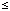x1 is self-similar to that on 1x3 after scaling. The proof is omitted here to save space. Most functions only approximate linear quality improvement. The area to bound is shown in Figure 1.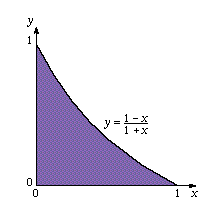Figure 1. Area to be bounded by HINT

At this point the reader may wonder at the fuss made over an integration. Why use hierarchical refinement with rigorous rational bounds instead of Gaussian quadrature, or at least Simpson's rule, with ordinary floating-point variables? First, we are trying to capture characteristics of many applications that use adaptive methods, including Barnes-Hut or Greengard algorithms for n-body dynamics, Quasi-Monte Carlo, and integral equations like those used for radiosity. Those methods find the largest contributor to the error and refine the model locally to improve answer quality. Second, benchmarks (and well-written applications) must have mathematically sound results. HINT, as defined above, has both characteristics in a concise form.

This task adjusts to the precision available, and has unlimited scalability: By this we mean that there is no mathematical upper limit to the quality that can be calculated, only a limit imposed by the particular computer hardware used (precision, memory, and speed). The lower limit is extremely low; about 40 operations yield a quality of about 2.0. A human can get that far in a few seconds. The quality attained is order N for order N storage and order N operations, so the scaling is linear. Maintenance of a queue of errors needs little pointer management. A simple one-dimensional data structure holds a pointer to the beginning (which should be the largest error) and the end (where new error information is placed). The program for HINT is available by Internet (see last section) for readers interested in specific details.

We illustrate by showing an ultra-low-precision HINT computation with eight-bit data. For a given word size of bd bits, the x and y axis will be represented by [ bd /2] and bd - [bd /2] size quantities. For example, an eight-bit byte conveniently represents values from 0 to 255, so it could represent a grid 16 by 16 on which the graph of the function is superimposed. The program avoids the need to represent the overflow value of 256. Two precisions are needed: the precision of the data used to count units of area above and below the function, and the precision of the indexing of the intervals. The index must have at least enough bits bi to specify any position in the x or y directions, which means bi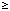bd - [bd / 2 ] bits. For eight-bit data, we need only four-bit indexes since there will be at most 16 subintervals.

If nx and ny are the numbers of area units in the x and y directions, and i is the number of the column, then (1- x)/(1+ x) can be computed as the fraction ny (nx - i) / (nx + i) divided by ny without overflow for all whole numbers i in the open interval (0, nx). Rounding the division in ny (nx - i) / (nx + i) up or down gives upper and lower bounds, respectively. For example, x = 1/ 2 is represented by i = 8. Then ny (nx - i) / (nx + i) is

16 * (16 - 8) / (16 + 8) = 128 / 24.

This last division makes the maximum use of the eight-bit precision, because the numerator takes all eight bits to express. This is the reason the numerator is scaled by ny. The quotient is 5 with remainder 8, so the function is bounded by

5 /16 < f (1/ 2) < 6 /16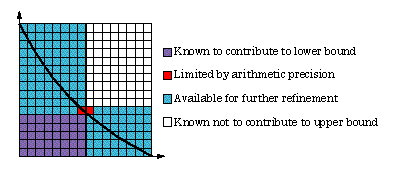Figure 2. Integration with byte-precision numbers, two subintervals

Figure 2 shows the state of the bounds after subdivision into two intervals. The areas in the upper left and lower right contain 87 and 47 squares, respectively. One square in each region is due to imprecision and cannot be eliminated by subdivision. To reduce the error, the 87-square region should be subdivided. The 47-square error will then move to the front of the queue of subintervals to be split.

A key idea of HINT is the use of whole number arithmetic to preserve the associative property. The need for associative arithmetic stems from the way the total error is updated. Whenever a subinterval is split, the error contribution of the parent subinterval is subtracted and the two smaller child errors added to the total error. This must be done without rounding, or else roundoff would accumulate as HINT runs.

For floating-point arithmetic, it is not generally true that (a + b) + c = a + (b + c). However, most machines can guarantee that this equality is true if the sum and intermediate sums are all whole numbers within the mantissa range. For example, 32-bit IEEE floating-point arithmetic effectively has 24 bits of mantissa. It can express the whole numbers

0, 1, 2, ..., 16777214, 16777215

exactly, much as 2's complement arithmetic can for an unsigned 24-bit integer. By restricting the computations in HINT to whole numbers, we can make use of any hardware for fast floating-point arithmetic. It is quite possible for the floating point hardware to be faster than the integer hardware, especially for multiplication. Yet, the same problem can be run with either type. By writing the kernel of HINT in ANSI C with extensive type declaration in the source text (including type casting every integer that appears explicitly), we need only change the preprocessor variable DSIZE from float to long to run HINT for the two data types! We are not aware of this degree of portability having been achieved in other programs.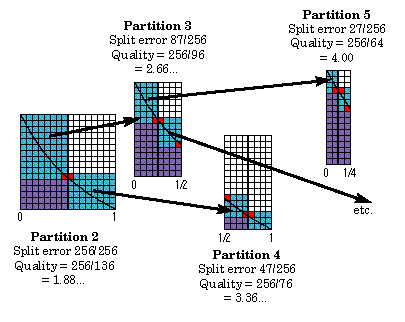Figure 3. Sequence of hierarchical refinement of integral bounds

By tracking the total error in this manner, a scalar can record the total error at any time without requiring an order N traversal of the tree. The control structure of HINT is explicitly order N for N iterations, and HINT makes steady progress to quality that is order N. Thus, a computer with twice the QUIPS rating can be thought of as "twice as powerful"; it must have more arithmetic speed, precision, storage, and bandwidth to reach that rating.

If there were no loss of precision, with each function value exactly representable on the computer, the Quality would always equal the number of the partition. The decision about which subinterval to split next takes into account the squares lost through insufficient precision. Finding the error that can be removed is not just a matter of multiplying the width by the difference between upper and lower bounds and then subtracting the two corners. When the width becomes one square or the upper and lower bounds differ by less than two squares, nothing is gained by refinement. This exception is easily handled by computing with boolean variables and need not involve explicit conditional branches that often degrade performance. Ultimately, there is no error left that can be eliminated by subdividing intervals. The HINT run then terminates with an "insufficient precision" condition. Figure 4 shows the limit of an 8-bit precision computation.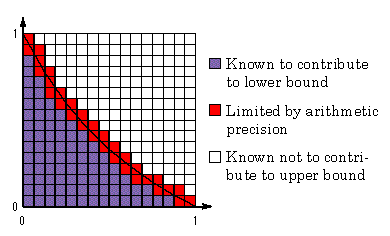Figure 4. Precision-limited last iteration, 8-bit data

### 2.2 Memory and operation requirements

While it is possible to do integration with little more memory than an accumulator and a few working registers, the goal of steady progress toward improved quality means we must compute and store a record of each upper-lower bounding rectangle. The main data structure of HINT is the record describing a subinterval. It contains the left and right x values xl and xr, the upper and lower bounds on the function of those values, the number of units in the upper and lower bounds, and the width of the interval (to avoid recomputation).

If bd is the number of bits required for a data quantity and bi is the number of bits required for an index, then the storage required for n iterations is (9bd + 4bi)n bits. Similar measures apply for non-binary computers; simply replace "bits" with digits in whatever number base is used. For example, a vintage 1978 minicomputer with 4-byte floating-point data and 2-byte indexing would take (9 x 4 + 4 x 2)n = 44n bytes for the data. [Program storage varies widely, but HINT is not designed to exercise the handling of large program executables. Users of programs believed to stress instruction caching should not use HINT as a performance predictor.]

By traditional "flop" counts using methods like those suggested by McMahon  (a divide counting as four floating-point operations, for example), each HINT iteration takes about 40 operations. This may seem high, but considerable work is expended rigorously computing the potentially removable error remaining in a subinterval. One is free to elect any data type, so a HINT iteration with 64-bit integers will measure no floating-point operations. Our initial experiments show that performance in QUIPS is remarkably similar for different data types on a computer, for comparable execution times; see Section 4.1. The "personality" of a computer is partially revealed by its higher performance using integer or floating-point data. A much higher performance for integer operations might reflect less hardware emphasis on scientific simulation and more on functions such as editing and database manipulation (i.e., business versus scientific computing).

A compilation of the HINT kernel for a conventional processor revealed the following operation distribution for indices and data:

 Index operations: Data operations: 39 adds or subtracts 69 fetches or stores 16 fetches or stores 24 adds or subtracts 6 shifts 10 multiplies 3 conditional branches 2 conditional branches 2 multiplies 2 divides

With a memory cost of about 20 to 90 bytes per iteration and an operation cost of about 40 operations per iteration, the ratio of operations to storage is roughly 1-to-1. For this reason, HINT reveals memory regimes and taxes bandwidth, a critical issue to accurate performance prediction. LINPACK , matrix multiply, and the Solver section of the original SLALOM benchmark have overly high ratios of operations to memory references. We maintain that mainstream computing is memory bandwidth limited and that most benchmarks disguise, rather than reveal, the limits of that bandwidth. We plan to correlate application performance with HINT measurements to verify that HINT accurately predicts application performance.

Many RISC workstations depend heavily on data residing in primary or secondary cache, and performance can drop drastically on large applications that do not cache well. The largest vector computers are fast within the confines of undersized static-RAM memories, but must use disk I/O or SSD-type storage to scale execution times up to what people are willing to wait. Paging to disk, for computers that support it, is clearly visible in HINT speed graphs as a steep drop in performance between two regions of relatively constant QUIPS (see Section 4).

### 2.3 Parallel versions

Parallel computing is now pedestrian enough that a number of hardback books on it are available at an introductory level. Some of these (see [7, 9]) use as a simple example the task of integrating 4 / (1+ x2) from 0 to 1 by simply partitioning the [0,1] interval among the processors. Since the analytical answer is pi, one gets the tutorial satisfaction of comparing the program output to 3.14159.... We believe credit is due Cleve Moler for introducing this example as a tutorial while he was on the staff of Intel Corporation. While a HINT benchmark could use 4 / (1+ x2) for its function, we arrived at (1- x) / (1+ x) instead because it favors neither x nor y decompositions, can be computed using fixed point (integer) arithmetic without overflow using the maximum representable whole numbers, and yields a theoretical quality Q = N after N hierarchical subdivisions.

To make HINT run in parallel, one need only make a few alterations to the approach described for the p calculation. In the textbook examples, each processor is responsible for a single subinterval of [0,1]. For instance, processor j of p processors might integrate the interval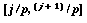. The processors then consolidate their partial sums. We modify this in that we integrate a different function, use precise whole-number upper-lower bounds, and use a moderate amount of scattered decomposition in the interval. We let each processor take a sampling of scattered starting intervals, not a single interval. Too many starting intervals increases time to reach the first answer. Too few decreases the ability of each processor to pick the best interval to split, and a characteristic "scallop" formation occurs in the graph of QUIPS versus time as processors make slightly less effective choices about where to concentrate their splitting efforts. We use the compromise of four scattered intervals, but this is user-adjustable.

Measuring the performance of parallel computing has been especially difficult because the source programs must be altered, and because most benchmarks do not scale. HINT solves the first problem by making the kernel as small and as easy to parallelize as possible without sacrificing realism. The scalability and tolerance for varying memory sizes have already been explained. Thus, HINT can provide performance data for even the most exotic architectures in roughly the same amount of time and effort as a conventional benchmark on a conventional serial computer used to take.

### 2.4 Anticipated objections to HINT

No benchmark can predict the performance of every application.
Absolutely true. It is easy to find two applications and two computers such that their rankings are opposite depending on the application; therefore, any benchmark that produces a performance ranking must be wrong on at least one of the applications. We maintain, however, that memory references dominate most applications and that HINT is unique in its ability to measure the memory-referencing capacity of a computer. Our early tests indicate it has high predictive powers, much better than extant benchmarks; see Section 4.3.

It's only a kernel, not a complete application.
There is considerable difference between a kernel like dot product or matrix multiply and the problem of rigorously bounding an integral. Most "kernels" are code excerpts. The work measure is typically something like the number of iterations in the loop structure, or an operation count (ignoring precision or differing weights for differing operations). HINT, in contrast, is a miniature standalone scalable application. It accomplishes a petty but useful calculation, and defines its work measure strictly in terms of the quality of the answer instead of what was done to get there. Although each iteration is simple, it still involves over a hundred instructions on a typical serial computer, and includes decisions and variety that make it unlikely a hardware engineer could improve HINT performance without also improving application performance. HINT resembles a Monte Carlo calculation in that the calculation can be stopped at any time; for both HINT and Monte Carlo methods, the answer simply gets better with time.

QUIPS are just like Mflop/s; they are nothing new.
One can translate Whetstones to Mflop/s, SPECmarks to Mflop/s, and LINPACK times to Mflop/s. QUIPS measures something more fundamental, and no such translation is meaningful. A vector computer or a parallel computer will probably have to do more operations to equal the answer quality of a scalar or serial computer. Conventional benchmarking would credit the vector or parallel computer with every operation performed, without regard to the utility of the operation. We feel QUIPS is an improvement over MIPS and Mflop/s in this respect. Also, a computer can get a high QUIPS rating without performing a single floating-point operation, since one is free to use whatever form of arithmetic (integer, floating point, even character-based) suits the architecture. On a given computer, the quality improvements are not proportional to the number of operations once the limits of precision begin to show. QUIPS resemble Mflop/s in the "per second" suffix, but the resemblance ends there.

This will just measure who has the cleverest mathematicians or the trickiest compilers.
Unlike SLALOM and other benchmarks with liberal definitions, HINT is not amenable to algorithmic "cleverness." It is already order N, and the rules clearly forbid that any knowledge about the function being integrated is used, other than the fact that it is monotone decreasing on the unit interval. Similarly, common compiler optimizations are all that are useful. While there is a major improvement in using optimization over using no optimization, we haven't seen any way to improve the optimized output very much... even with hand-coded assembler.

For parallel machines, the only communication is in the sum collapse.
The "diameter" of a parallel computer is the maximum time to send a communication from one processor to another. This has much to do with the performance of algorithms that are limited by synchronization costs, global decisions (such as convergence criteria or energy balance), and master-slave type work management. Testing a sum collapse is an excellent way to get a quick reading of the diameter of a parallel computer. We challenge anyone to find a more predictive test of parallel communication that is this simple to use.

## 3. Single-number ratings: Net QUIPS

There is always a tug-of-war between the distributors of computer performance data and the casual interpreters of it. The distributors tend to produce copious data showing the different facets of the measurement, and the interpreters tend to want a single number that answers the question, "How good is it?" Anticipating that our graphs of QUIPS versus time or QUIPS versus memory size for various data types will be summarized, especially for marketing and procurements, we have defined a method of distilling a QUIPS graph down to a single number: Net QUIPS is the integral of the quality Q divided by the square of the time, from the first time of quality improvement t0 to the last time measured. This is the same mathematically as the area under the QUIPS curve, plotted on a log(time) scale.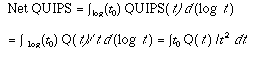Table 1 shows a SLALOM-style list of single-number QUIPS ratings. "fp" indicates 64-bit IEEE floating point, and "int" means the 32-bit integer QUIPS. All were run by Q. Snell and J. Korver at Ames Lab in June to September 1994, except for the Paragon SUNMOS runs which were done at Sandia by Q. Snell in September 1994.

If more (user-available) memory or cache is added to a system, then the QUIPS will be high for a larger range of time and thus improve Net QUIPS. Improved precision will lift the Q overall, and thus increase Net QUIPS. Lack of interruptions from dæmons or other users will be reflected with higher Net QUIPS. Lower latency will allow the integration to start with a smaller t value and can dramatically improve Net QUIPS. Burst speed and sustained speed are both reflected in Net QUIPS. Philosophically, Net QUIPS totals the QUIPS weighted inversely with the time it takes to get to that speed. The unit of Net QUIPS is quality improvement per second, the same as QUIPS.

We are hoping people will learn to interpret HINT graphs like those shown in Section 4, and not have to rely on single-number distillations. We have not included the peak Mflop/s ratings of the computers in Table 1, feeling that they fail to convey any useful information in most cases and often mislead.

Net QUIPS can be used to compare two operating systems, as shown by the 32-processor Paragon entries for SUNMOS and OSF shown in Table 1. It also can be used to make speedup plots, although we feel "speedup" is another misleading metric as typically measured and reported. A cursory examination of the table shows that Net QUIPS does not quite double when the number of processors doubles, yet the performance scales over a wide range. This corresponds well to studies of practical applications measured using scaled speedup. It is possible to measure the speed of humans using paper and pencil with HINT. Our initial experiments with college-educated adults indicate that a person is about 0.1 QUIPS. People, unlike computers, tend to increase precision dynamically. HINT can allow one to make reasonable comparisons of the numerical computing power of humans compared to machines.

## 4. Examples

For the following HINT plots, we use a logarithmic scale for time, with approximately decibel resolution (10 divisions per decade) samples of the time axis. This usually has the effect of removing performance drops caused by occasional interrupts, but some performance discontinuities are repeatable functions of the architecture.

### 4.1 SGI Indy SC-various data types

To demonstrate the precision-independence of HINT, we ran it on a Silicon Graphics Indy SC for C types double, float, int, and short. These represent 53, 24, 32, and 15 bits of useful precision. See Figure 5. For regions where all four graphs are defined, the QUIPS are in a range ±15% of their mean value. The short run ran out of precision in a millisecond, but otherwise resembled performance for the other data types. The float and int runs were also precision-limited, not memory-limited. There is a characteristic decrease in quality improvement by about half near the end of a precision-limited run. The double run extended to the end of virtual memory.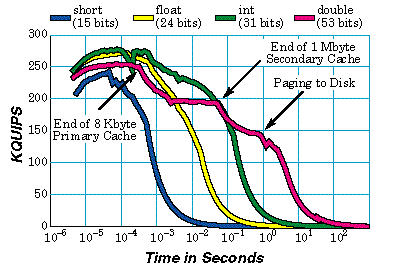Figure 5. Comparison of Different Precisions

Figure 5 indicates the presence of a primary and a secondary cache. Although the graph uses time as the horizontal axis, a graph using memory as the independent variable shows the dropoffs to occur at 8K bytes and 1M bytes. These are the data cache sizes on the SGI Indy SC.

### 4.2 Current workstations

The second example, shown in Figure 6, plots QUIPS for a variety of current workstations. It is interesting to note that the SPEC performance appears to be well predicted by examining the speed ratio in the 0.001 to 0.01 second range. Being a fixed-size benchmark, SPEC is doomed to periodic resizing and revision as advances in computer power make the old benchmark a mismatch to the computer capabilities. Since the current SPEC is already dated, it is not surprising that it now fits comfortably into the secondary cache of some workstations.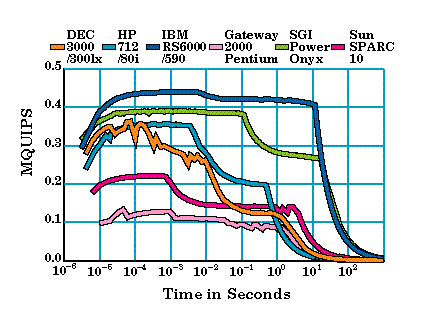Figure 6. Comparison of Various Workstations

### 4.3 Parallel computers

Systems at Ames Laboratory, Sandia National Laboratories, and Silicon Graphics were tested to obtain the graphical data shown in Figure 7. The number of processors for each is shown in the legend in parentheses.

The ratio of Intel Paragon QUIPS to those of the nCUBE 2S corresponds closely to the 2-4x performance per processor we have observed on applications. For example, the NAS Parallel Benchmarks [1, 3] show an overall performance (Cray Y-MP = 1.0) of 0.94 for 128 nCUBE 2S nodes, 1.61 for 256 nCUBE 2S nodes, and 2.19 for 128 Paragon nodes. The ratio per processor is consistent across all components of the NAS benchmark. [Note: Paragon nodes are about 8x the cost and form factor of nCUBE nodes.] The NAS benchmarks require man-months to port and tune to a particular architecture, and then run fairly on only a limited range of a parallel product line because of their fixed size. Since HINT provides similar information in less than two hours of conversion effort and runs on any size computer, we feel it is a more cost-effective and flexible way of obtaining predictive data for new architectures.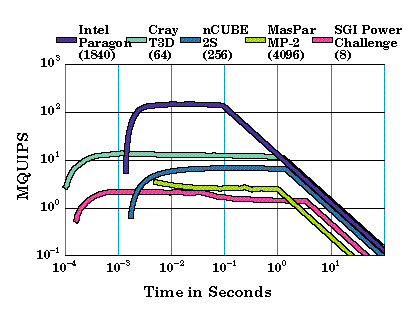Figure 7. Comparison of Several Parallel Systems

The "peak Mflop/s" rating of the Intel i860XP node is over 25 times that of the nCUBE 2S processor (75 Mflop/s versus 2.9 Mflop/s). This is an utterly misleading specification. Unless quad load instructions are used, the memory bandwidth is 200 MB/s for the Intel; this compares with 100 MB/s for the nCUBE. Hence, bandwidth seems to be the better raw specification to use, if one cannot perform a benchmark or application test. HINT reflects bandwidth. For the MIMD parallel computers, there is an "acceleration" up to the peak speed caused by the diameter of the ensemble, the amount of time to do global communication.

The MasPar and Intel computers exhibit the narrowest time range of any computers we have tested. Although their Net QUIPS ratings are respectable, their relatively long time to first result and short time before memory is exhausted imply they are special-purpose computers. Performance could be improved on the low end by complicating the program to use a subset of the processors, and possibly on the high end by using parallel I/O explicitly to extend storage. A narrow HINT graph indicates a special-purpose computer, probably caused either by unusually high latencies or insufficient memory relative to the computing speed.

## 5. Conclusions

The HINT benchmark is designed to last. It allows fair comparisons over extreme variations in computer architecture, absolute performance, storage capacity, and precision. It improves on SLALOM in being linear (answer quality, memory usage, and operations all are proportional), being very low cost to convert to different architectures, and unifying the precision and memory size into the performance. We have attempted to create a speed measure that is as pure and absolute as an information-theoretic measure can be, yet is practical and useful as a predictor of application performance. Time will tell whether HINT measures correlate well with the a wide variety of scientific applications; of course there will be applications for which HINT does not rank the computer-application combination correctly. However, we suspect it will predict application performance very accurately compared to other benchmarks now in use. Because HINT is simple and very easy to apply even on hard-to-use computer systems, we hope it will provide insight not otherwise available.

### References

This work is supported by the Applied Mathematical Sciences Program of the Ames Laboratory-U.S. Department of Energy under contract number W-7405-ENG-82.

Contact: John Gustafson john.gustafson@sun.com